Courses
Courses for Kids
Free study material
Free LIVE classes
More

# Addition and Subtraction for Class 4LIVE
Join Vedantu’s FREE Mastercalss

## Introduction to Subtraction and Addition Strategies

Hey kids! You might be aware of the concept of addition. But if you are not, no worries; we are here to teach you how to add different values using 4th-grade addition strategies. Addition and subtraction are arithmetic operations in mathematics that calculate the sum and difference between different operands, such as whole numbers, integers, fractions, algebraic expressions, and so on.## What are Addition and Subtraction?

The addition is done by summing up or putting things together. It is a way to combine numbers, operands, or objects. On the other hand, subtraction is done by taking away or removing things. It is a way to reduce numbers, operands, or objects.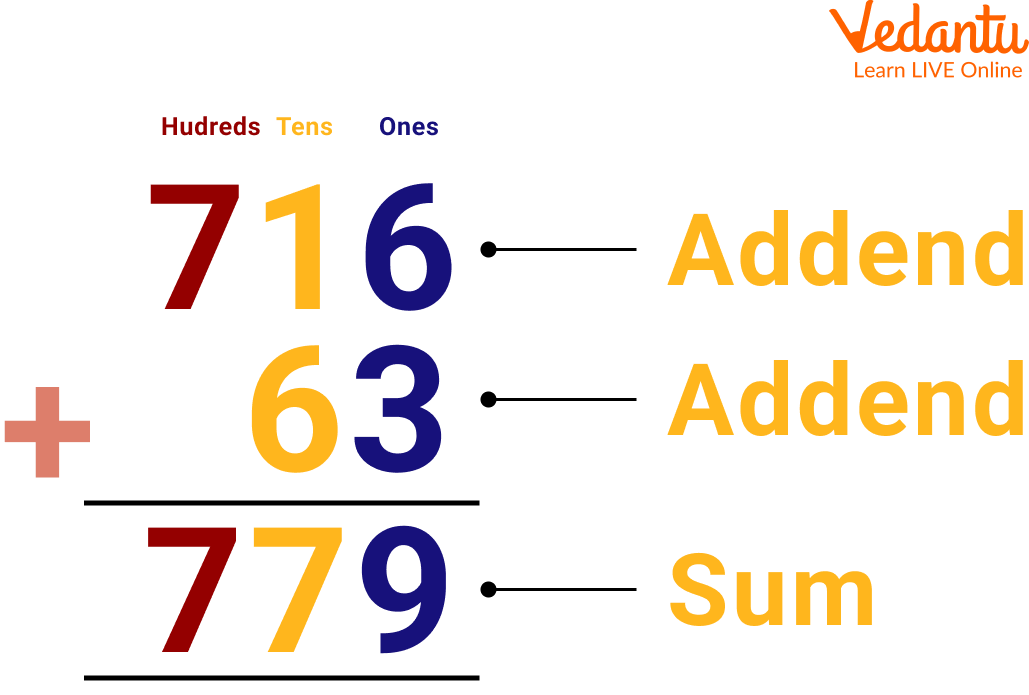The subtraction formula is stated as Minuend - Subtrahend = Difference. Here, minuend is the number from which the other number is subtracted, and subtrahend is the number that is subtracted from the minuend. The symbol (-) indicates the subtraction operation, and the difference indicates the result. The example given below shows the subtraction formula.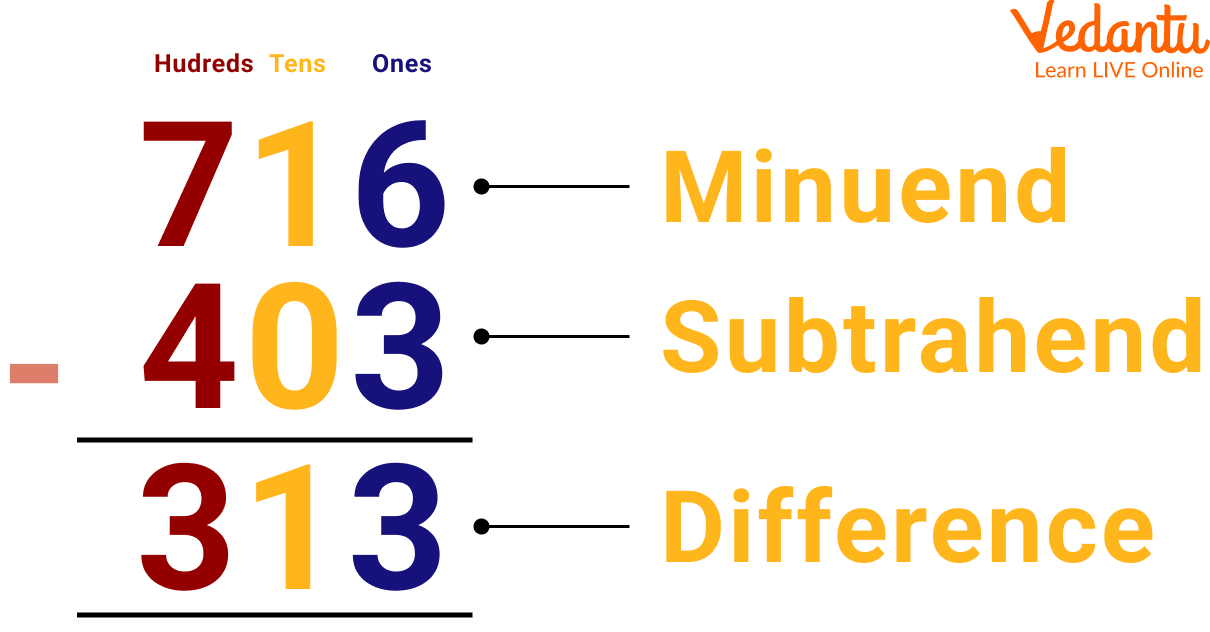Subtraction

In addition, there are two cases. One is a simple addition, in which, on adding the addend, the sum doesn’t exceed 9. This comes under the addition without regrouping. Another one is regrouping. It takes place whenever the sum of a column exceeds 9. Here, the extra digit is carried over to the preceding column and added along with the addends. In these two cases, we are going to study 4th-grade addition strategies with the help of an example. So let’s see them:

Example: Add the numbers 355 + 466.

Ans: Step 1: Start with the digits in one's column → 6 + 5 = 11. Here the sum is 11. The tens place digit of the sum (i.e., 1) will be carried over to the tens place.

Step 2: Then we will add the digits in tens place along with the carryover 1 → 1(carry-over) + 5 + 6 = 12. Here the sum is 12. The tens place digit of the sum (i.e., 1) will be carried to the hundreds column.

Step 3: Now, we will add the digits of hundreds place along with the carryover digit 1 → 1(carry-over) + 3 + 4 = 8. Here the sum is 8.

Step 4: Thus, 355 + 466 = 821

Below, the regrouping operation is shown for 355 + 466.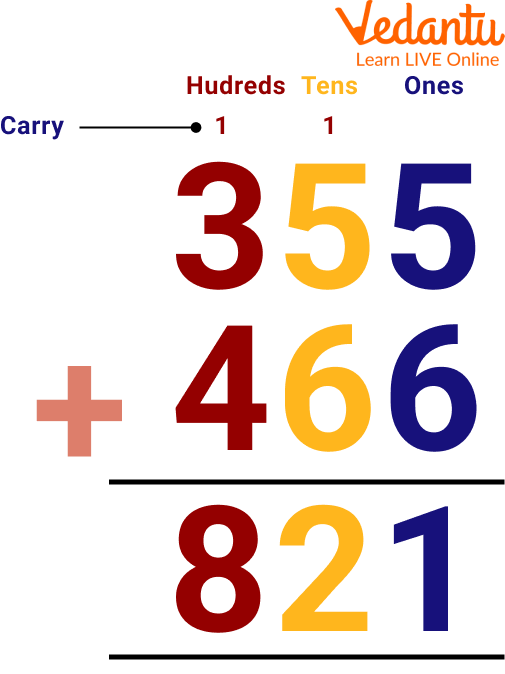Addition of Two Number with Regrouping

## Subtraction with Borrowing for Class 4

Subtraction with regrouping occurs whenever any digit in the minuend is smaller than the corresponding digit in the subtrahend. Here, we borrow 1 from the preceding column and combine it with this minuend so that this becomes greater than the subtrahend. Let us understand this with the help of an example.

Example: Subtract the numbers 432 - 256.

Solution: Let us use the following steps to subtract these numbers.

Step 1: Start with the digits in ones place. We see that 2 is smaller than 6. So, we will borrow 1 from the tens column, making it 12. Now, 12 - 6 = 6.

Step 2: After giving 1 to the ones column in step 1, the 3 in the tens column becomes 2. Now, let us subtract the digits in the tens place (2 - 5). Here, 2 is smaller than 5, so we will borrow 1 from the hundreds column. This will make it 12. So, 12 - 5 = 7.

Step 3: In step 2 we had given 1 to the tens column, so we are left with 3 in the hundreds place. We will now subtract the digits in the hundreds place, i.e., (3 - 2). So, 3 - 2 = 1.

Step 4: Therefore, the difference between the two given numbers is: 432 - 256 = 176.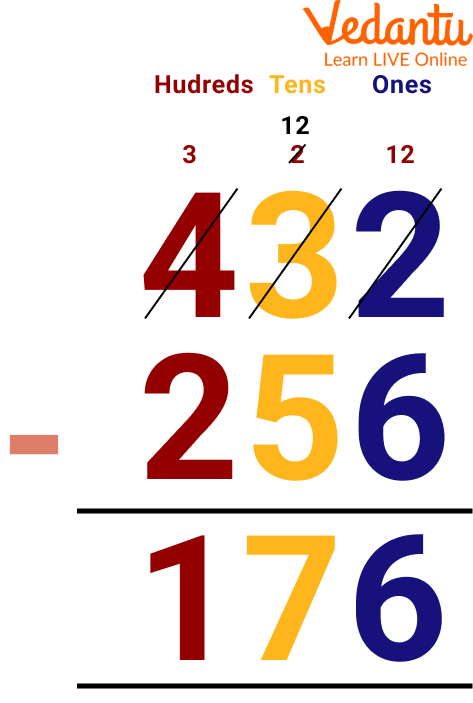Subtraction with Borrowing

## Solved Subtraction and Addition Examples for Class 4

Q 1 Anjali gave 51 toffees to Vijay and 28 toffees to Aman. How many toffees did she give?

Sol: Let's solve this problem with the regrouping method.

Total toffees Anjali gave to Vijal and Aman: 51 + 28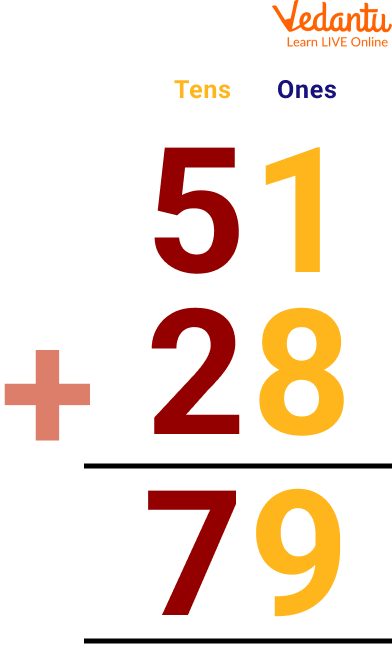Thus Anjali gave 79 toffee altogether.

Q 2 Aman got 20 marks in Maths out of 50 and 35 marks in English out of 50. How many marks he got in total

Ans: Marks in maths: 20

Marks in English: 35

Total marks 20 + 35 = 55

Thus, he got 55 marks in total.

Q 3 Rajni has 5 rupees, her father gave her 95 rupees, and her mother gave her 2 rupees. So how many rupees does Rajni have in total?

Ans: Rajni has 5 Rs

Her father gave her 95 Rs

Her mother gave her 2 Rs

So, Rajni and her mother's rupees equal, 5 + 2 = 7

Thus total amount becomes: 7 + 95

= Rs. 102

Therefore, the total amount that Rajni has is 102.

## Practice Problems of Addition and Subtraction for Class 4

Q 1. Jiya has 45 rupees in her pocket. She gave 34 rupees to her mother. How much money is left with her?

Ans: 11 rupees

Q 2. Add 45 and 23.

Ans: 68

Q 3. Sonu had 5 chocolates, her friend gave her 3 more chocolates, her father gave her 15 chocolates, and her mother gave her 29 chocolates. How many chocolates does Sonu have now?

Ans: 52

## Summary

To conclude, with the topic of 4th-grade addition strategies. We understood that addition is nothing but the combination of two or more two numbers. We add the digits to get a sum. Words problems are also solved with a simple method of addition. In this article, we also learned about addition with regrouping.

We solved some addition examples for class 4 and have practice problems of addition and subtraction for class 4 that need to be solved on our own. Now, we know how to add different numbers and can also add numbers using various addition strategies.

Last updated date: 27th Sep 2023
Total views: 78.6k
Views today: 0.78k

## FAQs on Addition and Subtraction for Class 4

1. What is the addition to the carrying method?

Addition with carrying methods is generally used for adding larger numbers. In this method, we put the numbers in rows and columns format and add the number on the right row first, if we get a number larger than 9 then we add the tens digit in the second row.

2. What is the commutative property of addition?

According to the commutative property of addition, if we change the sequence of the addition digits, then the sum will also remain the same.

3. When to use subtraction with borrowing for class 4?

Subtraction with borrowing for class 4 is used when any digit of the minuend is less than the subtrahend. Subtraction with borrowing for class 4 is considered to be a difficult topic, which can be made easy by practice.